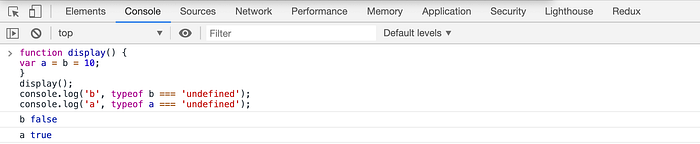# Yogesh Chavan# Tricky Javascript Code Snippets Asked In the Interview

Let's get started.

Predict the output of the below code:

``````const user = {
name: 'Raj',
location: {
city: 'NY',
state: 'NY'
}
};

const copy = Object.assign({}, user);
// OR
// const copy = { ...user };

copy.location.city = 'Albany';

console.log('original: ', user.location);
console.log('copy:', copy.location);
``````

The output of the above code is:

``````original:  {
city: 'Albany',
state: 'NY'
}
copy: {
city: 'Albany',
state: 'NY'
}
``````

The reason for the changed original object is because when we use `Object.assign` or `spread operator,` it only does `shallow copy`, which means while creating a copy of the object, properties of only the first level are copied

And if there are nested properties then only their reference is copied which means the copied reference still refers to the original place where the object is stored.

So in our case, the location property will still refer to the original object which is a user object in our case.

``````var number = 10;

var display = function () {
console.log(number);
var number = 20;
};

display();
``````

The output of the above code is not 10 but it’s undefined

Why?

This is because of hoisting in Javascript.

So the above code will be converted to the below code:

``````var number = 10;

var display = function () {
var number;
console.log(number);
number = 20;
};

display();
``````

As you can see only the declaration is moved to the start of the function and the value is not hoisted so the `console.log` prints `undefined` because `number` does not have any value assigned inside the function before the `console.log` statement.

``````const number = 1;

const result = (function () {
delete number;
return number;
})();

console.log(result);
``````

This code will not give any error because we are using delete on `number` but it will print the value 1 as the output.

This syntax of calling the function directly is known as IIFE (Immediately Invoked Function Expression).

The delete operator is used to delete the property of an object. Here, `number` is not an object but it's a primitive type so it will not throw an error but the function will return the original value of the variable which is 1 which is in the scope of the console.log statement.

``````const number = 1;

const result = (function (number) {
delete number;
return number;
})(10);

console.log(result);
``````

The output of the above code is 10.

In this code, we are passing the value 10 as the input to the function. But again inside the function number is just a local primitive type of variable so delete will not make any changes to the number and the value 10 passed to the function will be returned from the function.

``````function display() {
var a = b = 10;
}

display();

console.log('b', typeof b === 'undefined');
console.log('a', typeof a === 'undefined');
``````

The output of the above code is:

``````b false
a true
``````

This is because the assignment operator has a right to left associativity in Javascript which means it will be evaluated from right to left so first, b will be assigned the value 10 and then it’s assigned to a.

Therefore the following code,

``````function display() {
var a = b = 10;
}
``````

is same as

``````function display() {
var a = (b = 10);
}
``````

which is the same as

``````function display() {
b = 10;
var a = b;
}
``````

So b becomes a global variable because there is no var keyword before it and a becomes a local variable. Therefore, outside the function, only bis available so `typeof a === 'undefined'` comes as `true` and `typeof b === 'undefined'` comes as `false`.If we execute the above code in strict mode as shown below:

``````'use strict';

function display() {
var a = b = 10;
}

display();

console.log('b', typeof b === 'undefined');
console.log('a', typeof a === 'undefined');
``````

It will throw an error because b becomes a global variable and strict mode does not allow creating global variables so you will get an error when you execute this code.

Starting with ES6, there are many useful additions to JavaScript like

• ES6 Destructuring
• Import and Export Syntax
• Arrow functions
• Promises
• Async/await
• Optional chaining operator and a lot more.

You can learn everything about all the ES6+ features in detail in my Mastering Modern JavaScript book.

Check out free preview contents of the book here.

Also, you can check out my free Introduction to React Router course to learn React Router from scratch.

Want to stay up to date with regular content regarding JavaScript, React, Node.js? Follow me on LinkedIn.